# TIP4P-HB model of water

(Redirected from TIP4P-HB)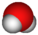This article is a 'stub' about water and/or ice. It has no, or next to no, content. It is here at the moment to help form part of the structure of SklogWiki. If you add material to this article, remove the {{Stub-water}} template from this page.
 rOH (nm)$\angle$HOH (deg)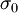$\sigma_0$O (nm)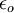$\epsilon_o$O (kJmol-1)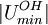$|U_{min}^{OH}|$(kJmol-1) qH (e)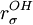$r_{\sigma}^{OH}$ (nm) rOM (nm)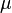$\mu$ (D) 0.09572 104.52 0.31536 0.6487 10.46 0.4415 0.14 0.015 1.85